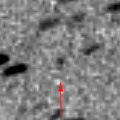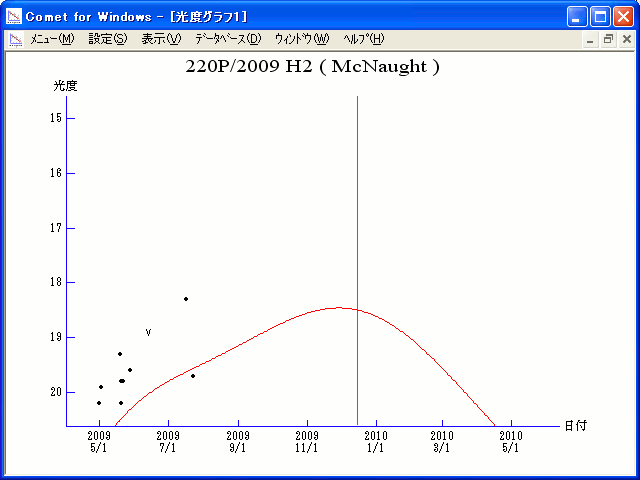# \$B%^%/%N!<%HWB@1(B

220P/McNaught (2009)

 English version Home page Updated on March 17, 2010###\$B%W%m%U%#!<%k(B

 \$BId9f(B 220P/2009 H2 \$B8!=PF|(B 2009\$BG/(B4\$B7n(B28\$BF|!"(B5\$B7n(B1\$BF|(B \$B8!=P8wEY(B 20.2\$BEy(B \$B8!=P T. H. Bressi (Spacewatch), G. Muler, J. M. Ruiz, and R. Naves (Lanzarate, Spain)

###\$B###\$B50F;MWAG(B

```                    Epoch = 2010 Jan.  4.0 TT
T = 2009 Dec. 15.43207 TT        Peri. = 180.75749
e = 0.5026812                    Node  = 150.11807 2000.0
q = 1.5486245 AU                 Incl. =   8.13271
a =  3.1139471 AU   n = 0.17936480   P =   5.495 years
```

###\$B8wEYJQ2=(B

```        m1 = 14.0 + 5 log\$B&\$(B + 15.0 log r
```##### \$B50F;MWAG\$O!"(BCBET 1790\$B\$K7G:\\$5\$l\$?\$b\$N\$G\$9!#(B \$B8wEY%0%i%U\$O(BComet for Windows\$B\$G:n@.\$7\$?\$b\$N\$G\$9!#(B